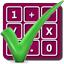#### Arithmetic of Computers

#### from Tenscope Limited

By using this site, you are accepting "session" cookies, as set out in the site's Privacy Policy
Cookies are also used to remember which page of the book you last viewed, so that when you revisit the site you automatically return to the last page you visited.

### Lesson 4

#### Page 125

Many ideas have been discussed in the last three lessons. You have acquired the tools and techniques you will need for handling other number systems. Now it’s time to review what you have learned.
We have had these definitions for cases where n is a positive whole number:
bn  = b × b × b × ⋯ × b (b used as a factor n times) b3  = b × b × b b2  = b × b b1  = b b0  = 1 ( provided b is not equal to 0) b − 1  = (1)/(b) b − 2  = (1)/(b2) b − n  = (1)/(bn)
How would you write 100 as a power of 10?